views 1017 words

## 为什么选择序列模型？（Why Sequence Models?）

Examples of sequence data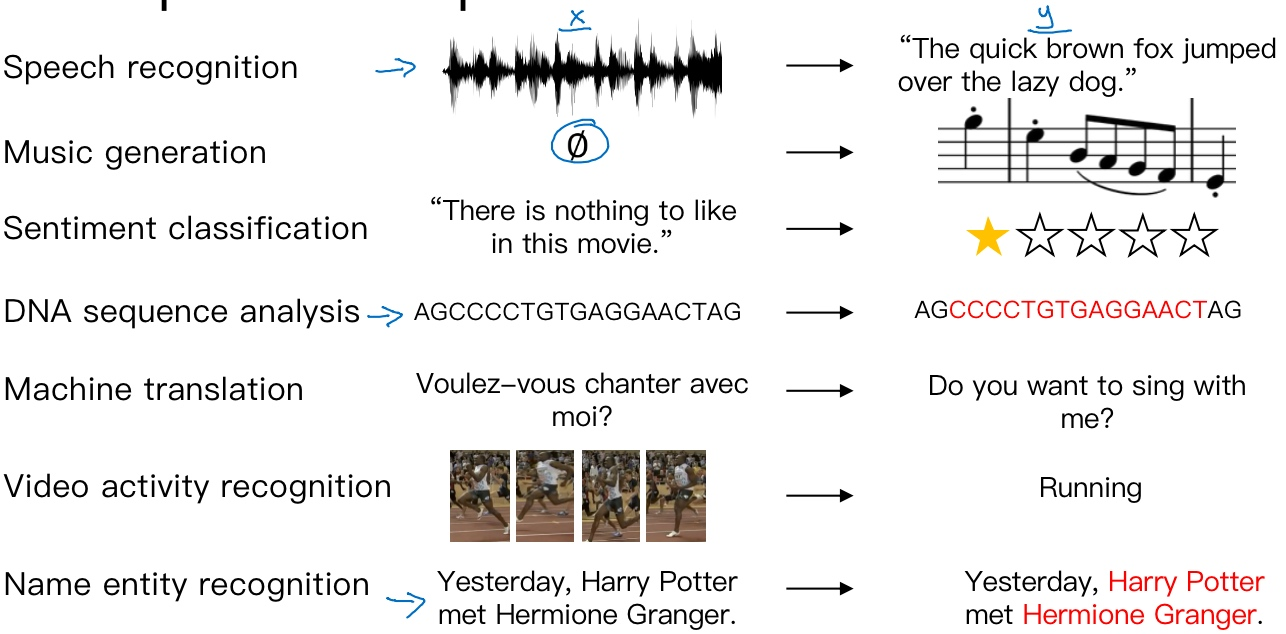• 输入数据X和输出数据Y都是序列，但X和Y有时也会不一样长
• 输入数据X和输出数据Y都是序列，X和Y有相同的数据长度
• 在另一些问题里，只有X或者Y是序列

## 数学符号（Notation）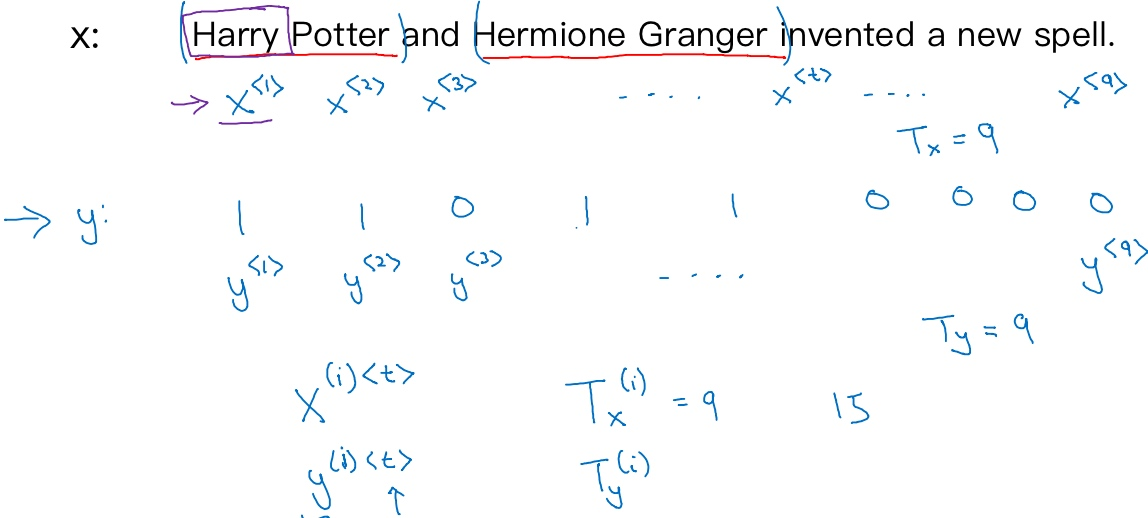t: temporal sequence, used to index the position in the sequence

$T_x = 9$: length of input sequence

$T_y = 9$: length of output sequence

$x^{(i)<t>}$: 训练样本i的序列中第t个元素

$T_x^{(i)}$: 第i个训练样本的输入序列长度

$y^{(i)<t>}$: 第i个训练样本的输出序列中第t个元素

$T_y^{(i)}$: 第i个训练样本的输出序列的长度

$x^{<t>}$的具体表示: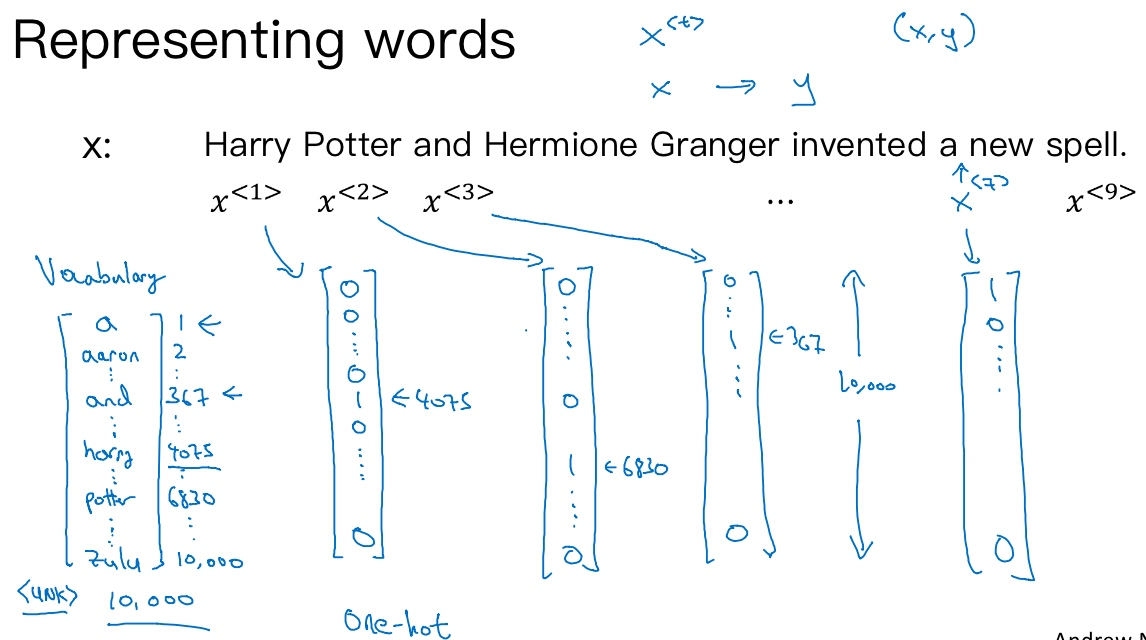• 每个词有不同的位置(index)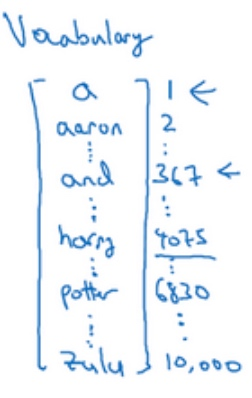• $x^{<1>}$表示Harry这个单词，它就是一个第4075行是1，其余值都是0的向量
• $x^{<t>}$指代句子里的任意词，它就是个one-hot向量，因为它只有一个值是1，其余值都是0，所以会有9个one-hot向量来表示这个句中的9个单词
• 目的是用这样的表示方式表示X，用序列模型在X和目标输出Y之间学习建立一个映射

## 循环神经网络模型 (Recurrent Neural Network Model)

Why not a standard network?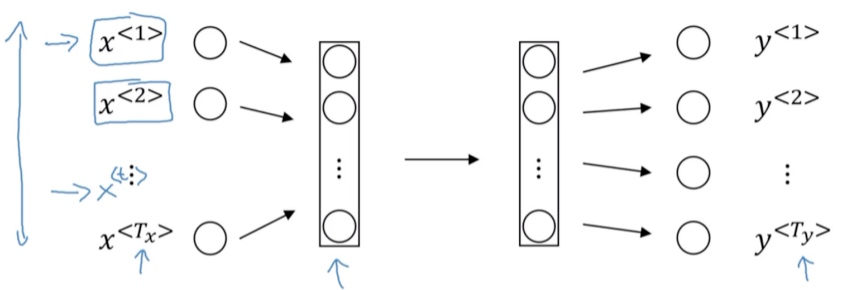Problems:

• Inputs, outputs can be different lengths in different example
• Doesn’t share features learned across different positions of text (具体来说, 如果神经网络已经学习到了在某个$x^{<t>}$, 即’Harry’可能是人名成分，那么如果Harry出现在其他位置，我们希望它也能够自动识别其为人名的一部分, 这是共享特征的结果, 如同CNN网络特点一样. 但是普通的神经网络不具备共享特征的能力)
• $x^{<1>},x^{<2>}, ..., x^{<t>}$每一个都是10000维的one-hot向量, 这是十分庞大的输入层, 因此第一层的权重权重将会有巨量参数. 共享特征还有助于减少神经网络中的参数数量，一定程度上减小了模型的计算复杂度.

Recurrent Neural Network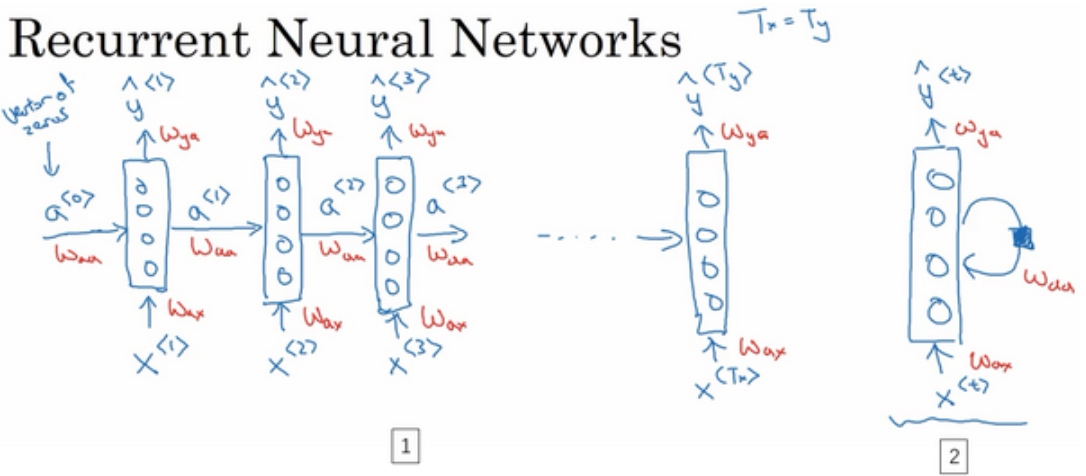• $W_{ax}$来表示管理着从$x^{<1>}$到隐藏层的连接的一系列参数, 每个时间步使用的都是相同的参数$W_{ax}$
• 而激活值(水平联系)是由参数$W_{aa}$决定的，同时每一个时间步都使用相同的参数$W_{aa}$
• 输出结果由$W_{ya}$决定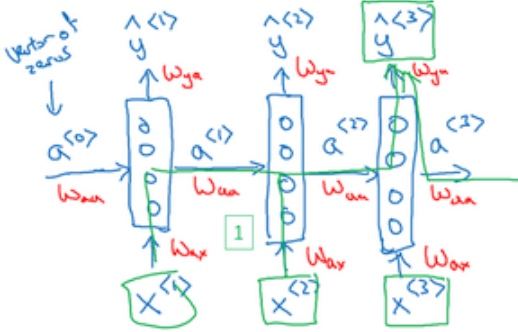• He said, ‘Teddy Stephsion was a good man’
• He said, ‘Teddy bears are on sale’
• 如果只给定前三个单词，是不可能确切地知道Teddy是否是人名的一部分，然而第一个例子是人名，第二个例子就不是.

• 双向循环神经网络 - bidirectional RNN（BRNN）

Simplified RNN notataion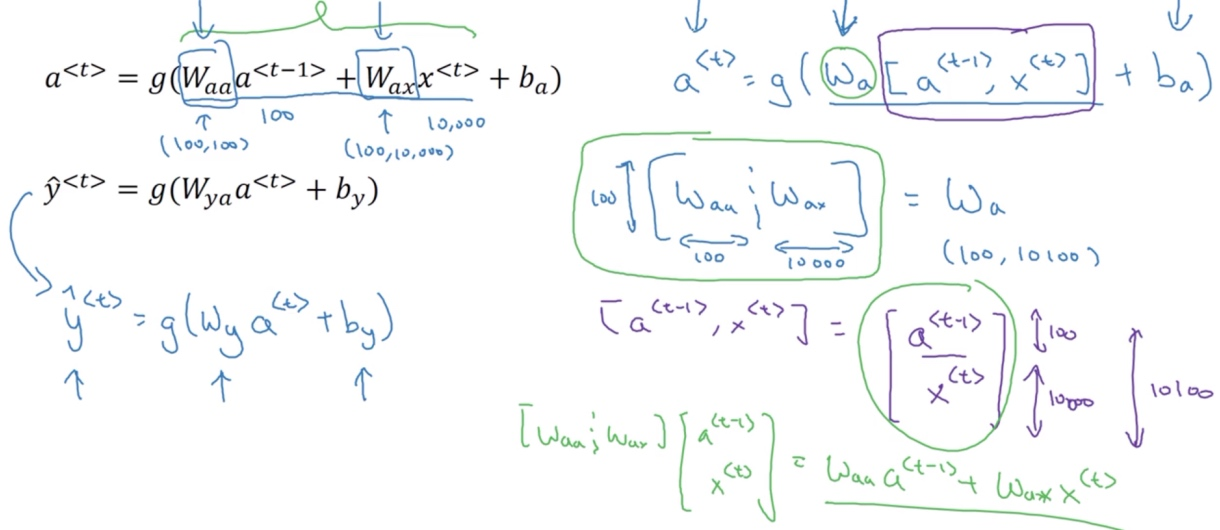• $a^{<1>} = g_1(W_{aa}a^{<0>} + W_{ax}x^{<1>} + b_a)$
• $\tilde{y}^{<1>} = g_2(W_{ya}a^{<1>}+b_y)$
• 通常$g_1$用的激活函数是tanh / ReLU
• 通常$g_2$用的激活函数是sigmoid / softmax

RNN的正向传播（Forward Propagation）过程为:

• $a^{<t>} = g_1(W_{aa}a^{<t-1>} + W_{ax}x^{<t>} + b_a)$
• $\tilde{y}^{<t>} = g_2(W_{ya}a^{<t>}+b_y)$

• $a^{<t>} = g_1(W_{a}[a^{<t-1>},x^{<t>}] + b_a)$
• $\tilde{y}^{<t>} = g_2(W_{y}a^{<t>}+b_y)$

• 如果a是100维的, x是10000维的, 那么$W_{aa}$就是个(100, 100)维的矩阵, $W_{ax}$就是个(100, 10000)维的矩阵. 将两个矩阵堆起来, $W_a$就会是个(100, 10100)维的矩阵.

$[a^{<t-1>},x^{<t>}]$就是将两个向量堆在一起, 即$\begin{bmatrix} a^{<t-1>} \\ x^{<t>}\end{bmatrix}$, 最终这就是个10100维的向量

RNN前向传播示意图：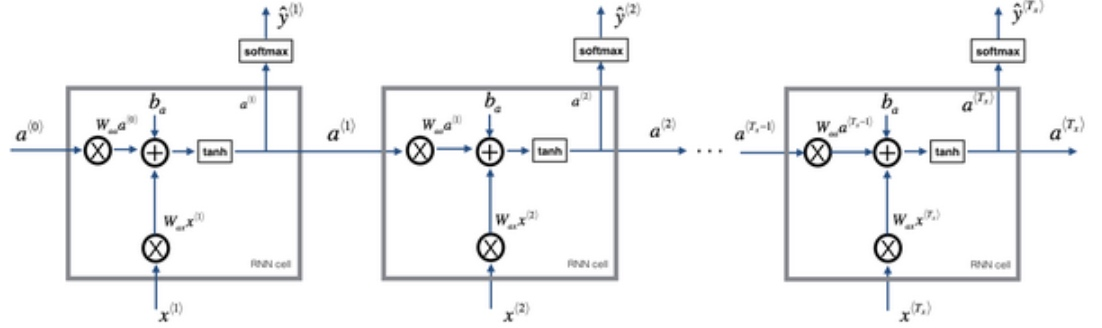### 例子## 通过时间的反向传播（Backpropagation through time）

Forward propagation and backpropagation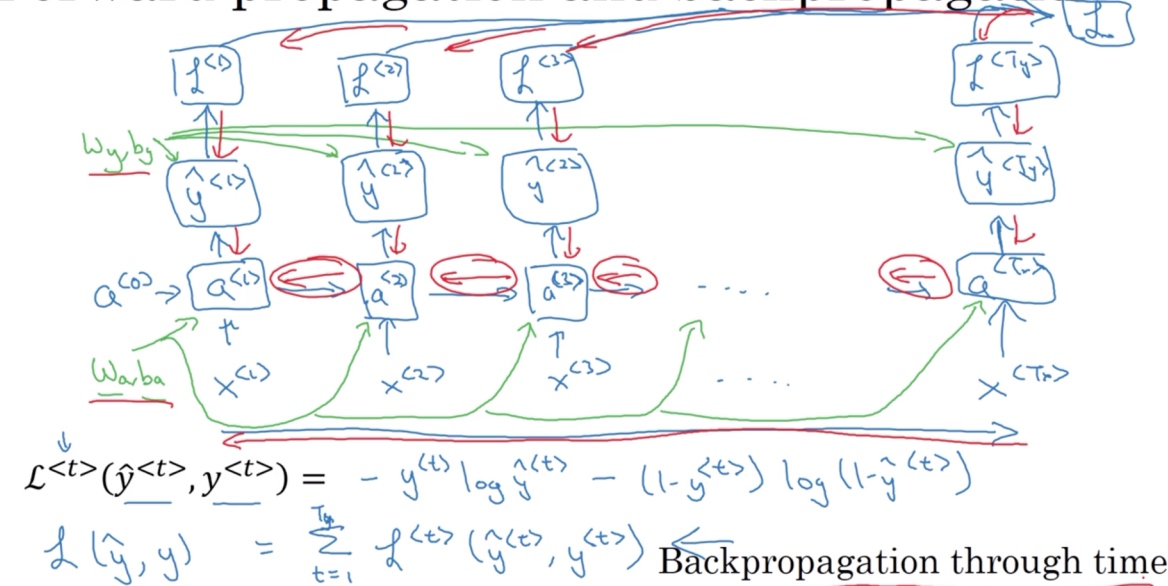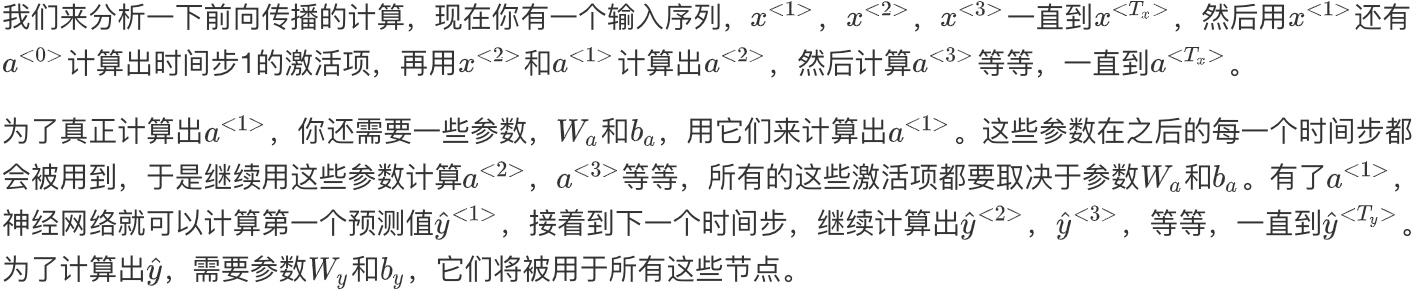RNN反向传播示意图: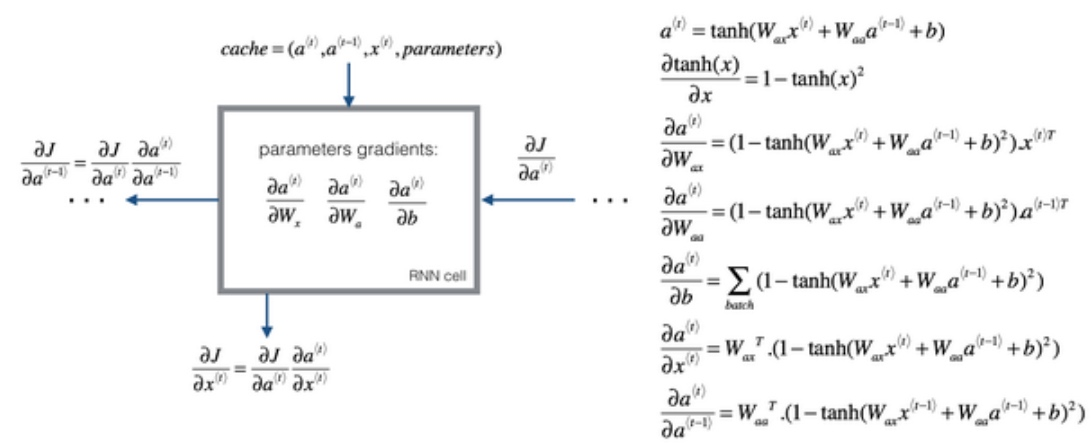## 不同类型的循环神经网络（Different types of RNNs）

Summary of RNN types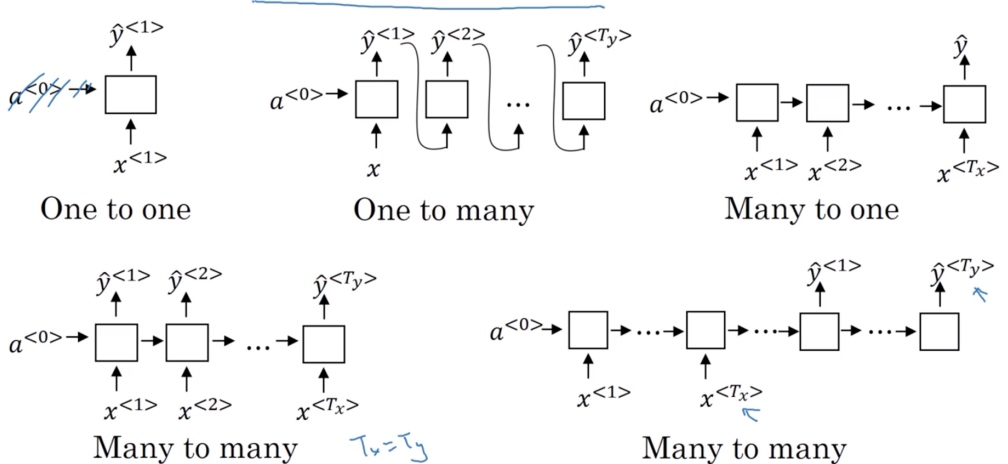One to One:

• When remove $a^{}$, it is a standard neural network

One to Many: - Music generation / sequence generation - $T_x$ may be 1 or $\emptyset$, E.g. input one genre of music that you would like to generate - When you are generating sequences, often you feed the first synthesized output to the next layer

Many to One:

• Sentiment analysis
• Input comments, output a rating number

Many to Many ($T_x = T_y$):

• Name entity recognition

Many to Many ($T_x != T_y$):

• Machine translation
• encoder-decoder architecture
• attention model

## 语言模型和序列生成（Language model and sequence generation）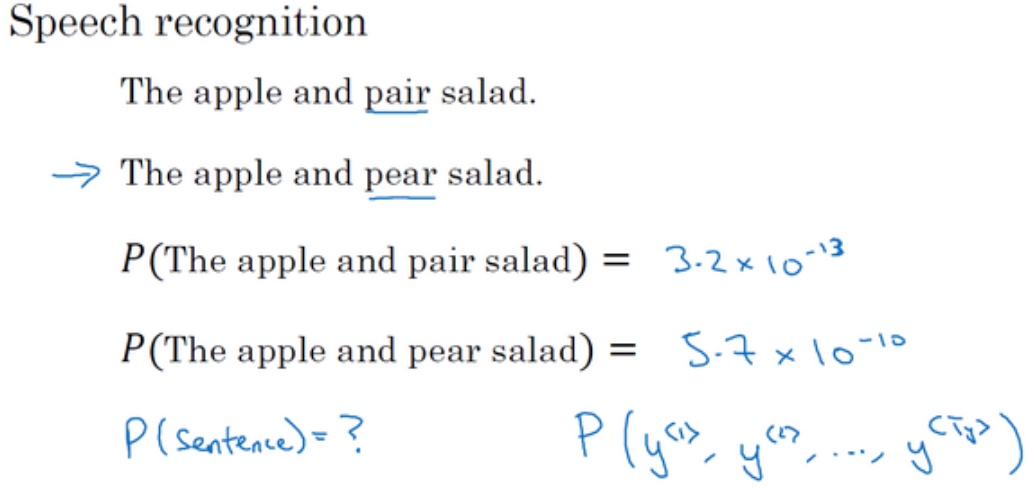‘The apple and pair salad’

‘The apple and pear salad’

language model: Given any sentence, it tells you what is the probability of that particular sentence (estimates the probability of that particular sequence of words)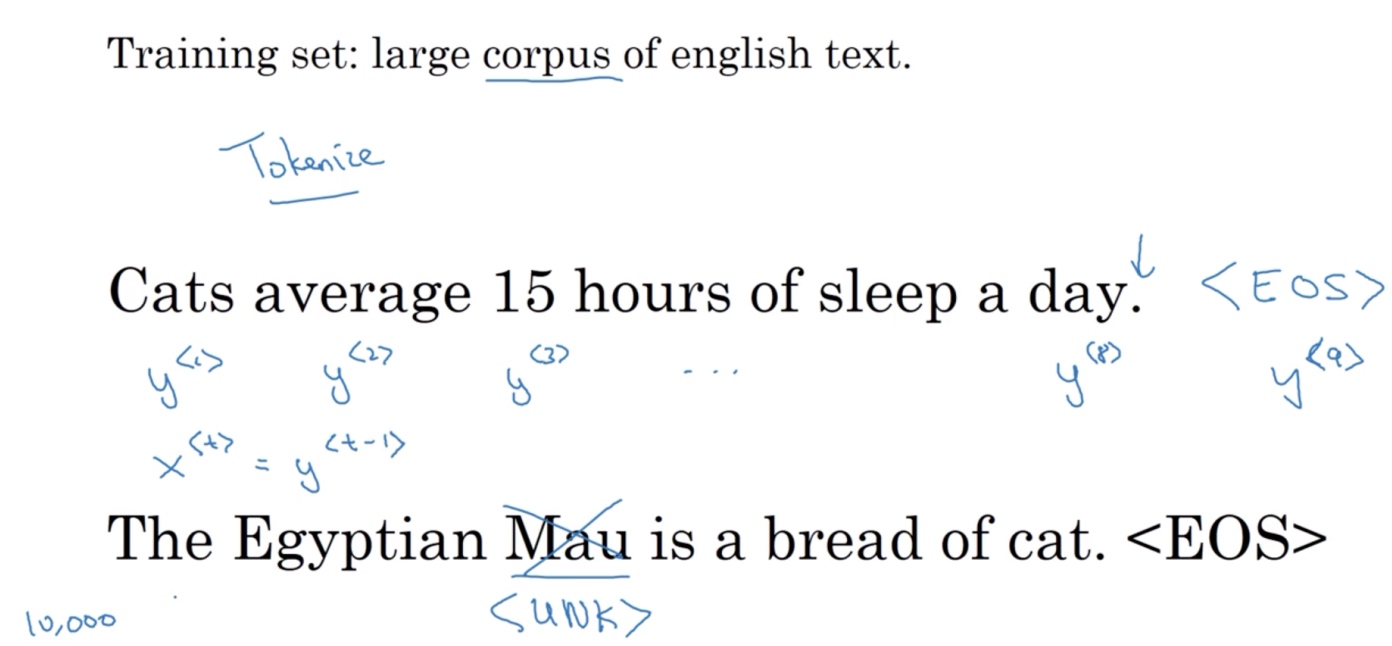#### 一. 标识化

‘Cats average 15 hours of sleep a day.’

‘The Egyptian Mau is a bread of cat.’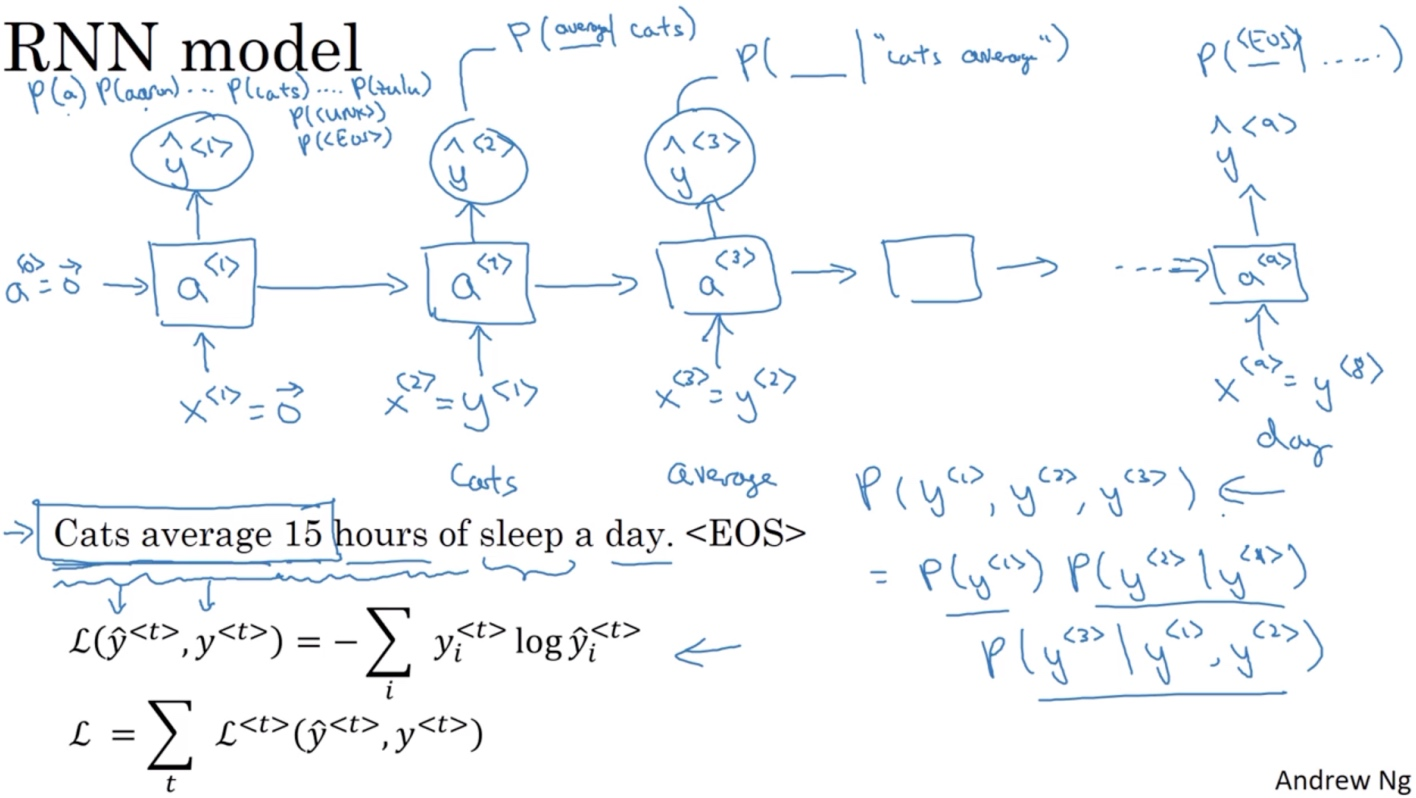#### 二. RNN建立序列的概率模型

• 在第0个时间步
• 计算激活项$a^{<1>}$，它是以$x^{<1>}$作为输入的函数, 而会被设为全为0的集合(0向量)
• 然后通过一个softmax层来预测字典中的任意单词会是第一个词的概率, $\tilde{y}^{<1>}$
• softmax层输出10,002种结果(每个词的概率), 因为字典中有10,002个词 $P(a), P(cats),P(<UNK>), ...,P(<EOS>)$
• 在第1个时间步
• 仍然使用激活项$a^{<1>}$
• 由于第一个词是’cats’的概率最大, 所以$x^{<2>} = y^{<1>}$
• 同样softmax层输出10,002种结果, $P(average|cats), ..., P(<EOS>|cats)$
• … 以此类推
• 所以$x^{<t>} = y^{<t-1>}$

#### 三. 代价函数

• 在某个时间步t, 如果真正的词是$y^{<t>}$，而神经网络的softmax层预测结果值也是$y^{<t>}$
• 那么softmax损失函数就是$L(\tilde{y}^{<t>},y^{<t>}) = -\sum_iy_i^{<t>}log\tilde{y}_i^{<t>}$
• 而总体损失函数就是$L= \sum_tL^{<t>}(\tilde{y}^{<t>},y^{<t>})$ (把所有单个预测的损失函数都相加起来)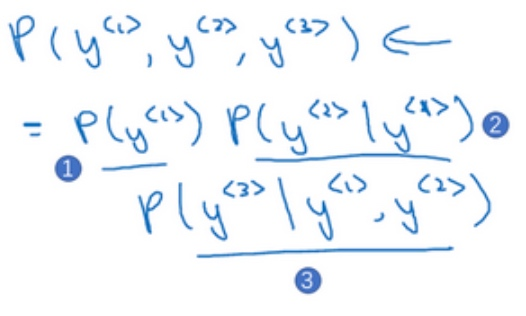• 第一个softmax层会告诉你$y^{<1>}$的概率, 这也是第一个输出
• 然后第二个softmax层会告诉你在考虑$y^{<1>}$的情况下$y^{<2>}$的概率
• 然后第三个softmax层告诉你在考虑$y^{<1>},y^{<2>}$的情况下$y^{<3>}$的概率
• 把这三个概率相乘，最后得到含3个词的整个句子的概率

### 对新序列采样（Sampling novel sequences）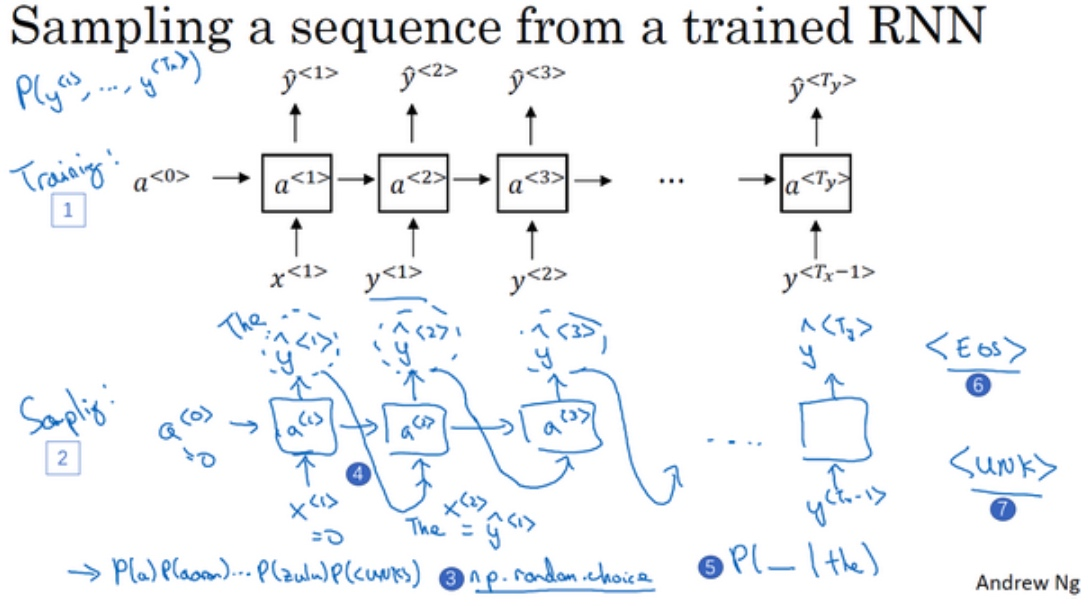1. 对模型生成的第一个词进行采样
• 输入$x^{<1>} = 0,a^{<0>} = 0$
• 对这个向量使用np.random.choice, 来根据向量中这些概率的分布进行采样, 这样就能对第一个词进行采样了
2. 继续下一个时间步,
• 需要$\tilde{y}^{<1>}$作为输入
• 然后softmax层就会预测$\tilde{y}^{<2>}$是什么
• 然后再次用这个采样函数来对$\tilde{y}^{<2>}$进行采样
3. 无论得到什么样的用选择结果(one-hot码表示的)，都把它传递到下一个时间步，然后对第下一个词进行采样，一直这样直到最后一个时间步
4. 这就是你如何从你的RNN语言模型中生成一个随机选择的句子

def choice(a, size=None, replace=True, p=None) - 表示从a中随机选取size个数 - replacement 代表的意思是抽样之后还放不放回去，如果是False的话，那么通一次挑选出来的数都不一样，如果是True的话， 有可能会出现重复的，因为前面的抽的放回去了。 - p表示每个元素被抽取的概率，如果没有指定，a中所有元素被选取的概率是相等的。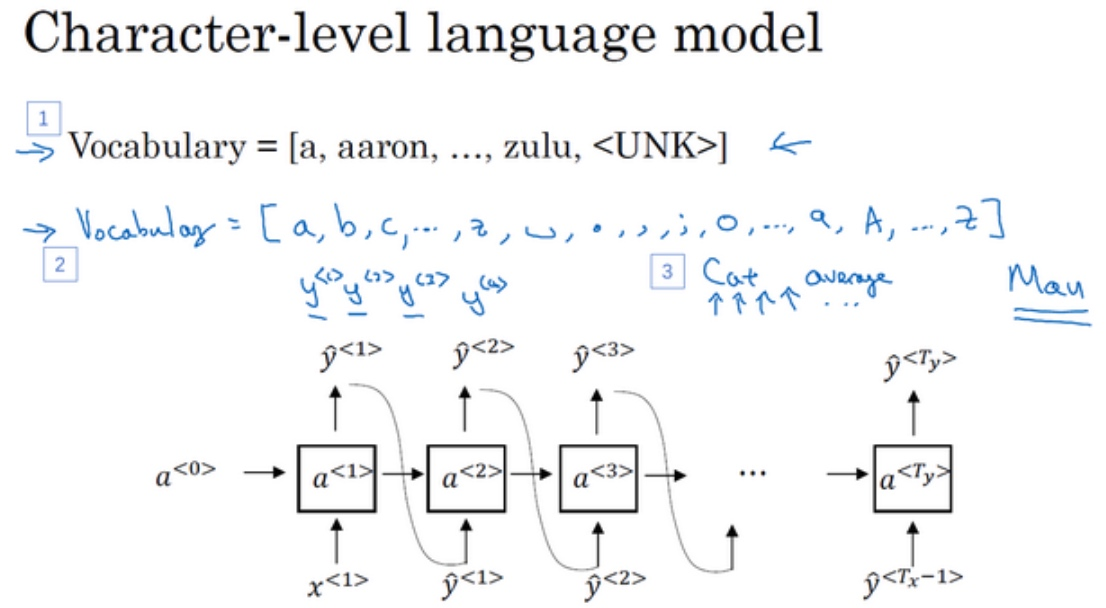Sequence Generation## 循环神经网络的梯度消失（Vanishing gradients with RNNs）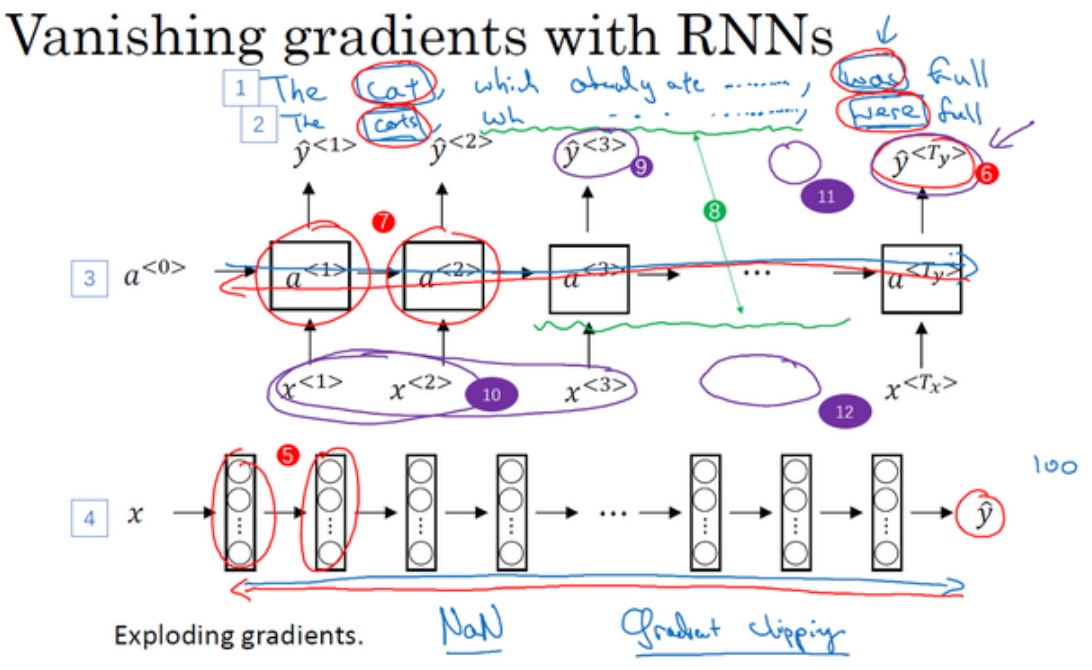‘The cat, which already ate ……, was full.’

‘The cats, which ate ……, were full.’Gated recurrent unit which is a very effective solution for addressing the vanishing gradient problem and will allow your network to capture much longer range dependencies. It is a modification to the RNN hidden layer that makes it much better capturing long range connection.

## GRU单元 (Gated Recurrent Unit)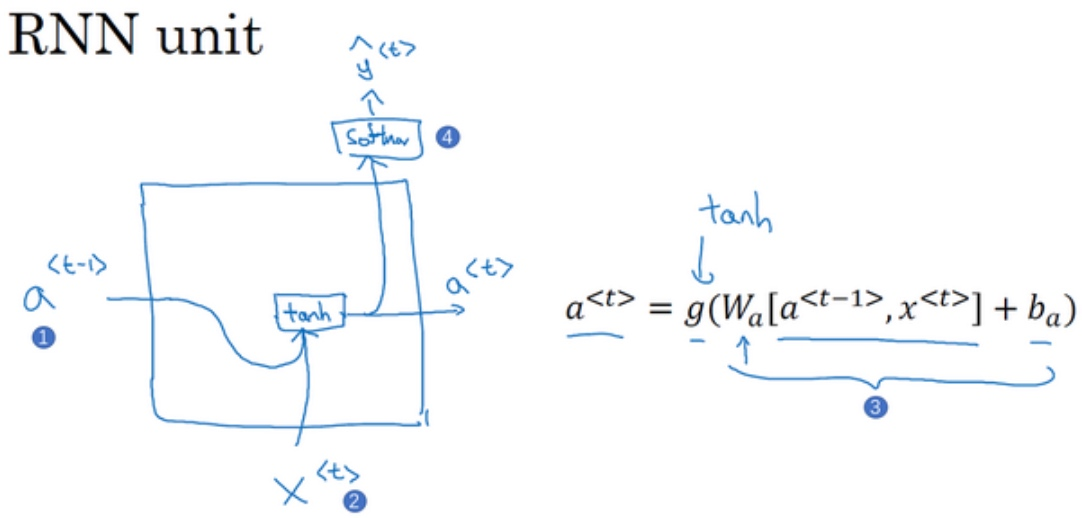$a^{<t>} = g(W_{a}[a^{<t-1>},x^{<t>}] + b_a)$在RNN的时间t处，计算激活值. 具体来看, 输入$a^{<t-1>}$(上一个时间步的激活值), 再输入$x^{[t]}$, 把这两个并起来后乘上权重项, 在这个线性计算之后, 经过tanh计算得到激活值$a^{<t>}$. 激活值将会传softmax单元(或者其他用于产生输出的东西).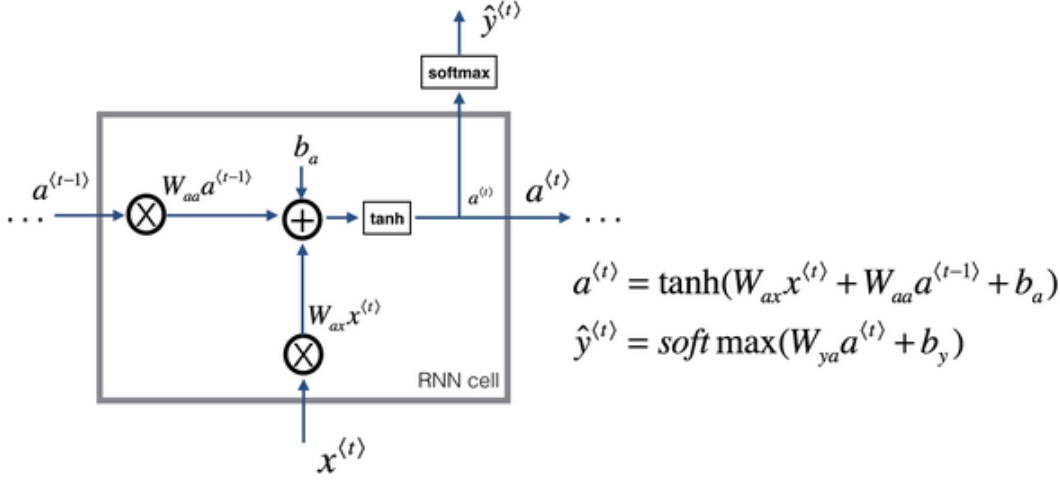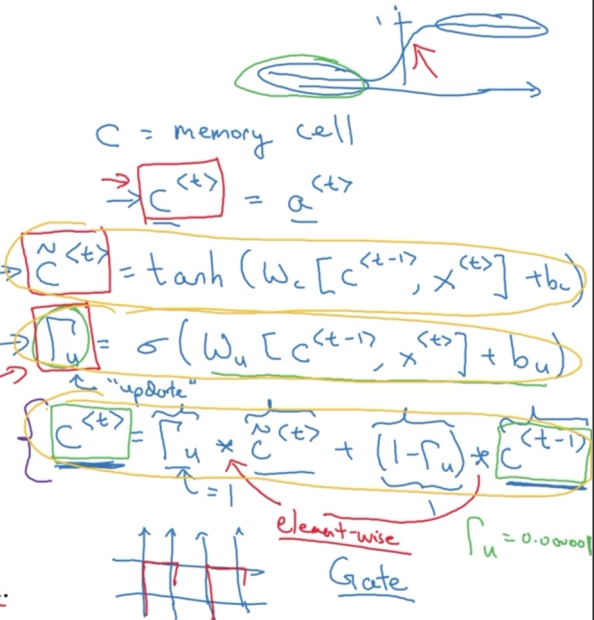1. GRU单元将会有个新的变量称为c (memory cell). 记忆细胞的作用是提供了记忆的能力, 比如说一只猫是单数还是复数, 所以当它看到之后的句子的时候, 它仍能够判断句子的主语是单数还是复数. 对于简单版本的GRU, 实际上它输出了激活值$a^{<t>}$, $c^{<t>} = a^{<t>}$
2. 在每个时间步, 考虑一个候选值$\tilde{c}^{<t>}$去重写记忆细胞(代替$c^{<t>}$的值). 用tanh激活函数来计算$\tilde{c}^{<t>} = tanh(W_c[c^{<t-1>}, x^{<t>}]+b_c)$
3. GRU中真正重要的思想是有一个门, $\Gamma_u = \sigma(W_u[c^{<t-1>}, x^{<t>}]+b_u)$
• u代表更新门, 值在0 - 1之间(因为用了sigmoid函数), 对于大多数可能的输入, sigmoid函数的输出总是非常接近0或者非常接近1.
• 门决定是否要真的要用$\tilde{c}^{<t>}$更新$c^{<t>}$
4. 接下来要给GRU用的式子, $c^{<t>} = \Gamma_u * \tilde{c}^{<t>} + (1 - \Gamma_u)*c^{<t-1>}$
• ‘*’ is element-wise multiply
• $\Gamma_u = 1$时, $c^{<t>} = \tilde{c}^{<t>}$

“The cat, which already ate……, was full.”

“The cats, which already ate……, were full.”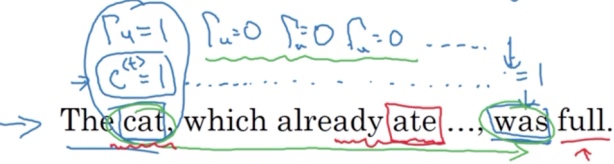1. 在’cat’位置的时候, 记忆细胞$c^{<t>}$将被设定为0或者1 (单数为1,复数为0), GRU单元将会一直记住$c^{<t>}$的值; 同时, 将门值$\Gamma_u$设为1
2. 然后往前再更新这个值$\Gamma_u$, 对于所有在这中间的值, 门的值应该设为0 ($\Gamma_u=0$), 意思就是说不更新它，就用旧的值 (因为$\Gamma_u=0$, 则$c^{<t>}=c^{<t-1>}$, $c^{<t>}$等于旧的值).
• 直到’was’的位置, $c^{<t>}$的值还是1 ($c^{<t>}$的值还是等于$c^{<t-1>}$), 于是它仍然记得猫是单数的, 所有用了’was’.
• $\Gamma_u$的作用就是决定什么时候会更新$c^{<t>}$值,
3. 到句子遍历完, 就可以忘记$c^{<t>}$值了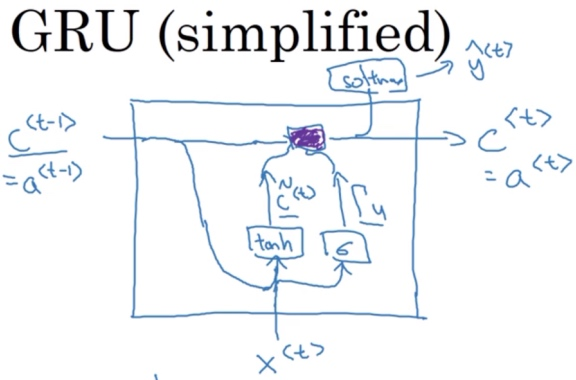GRU的图像表示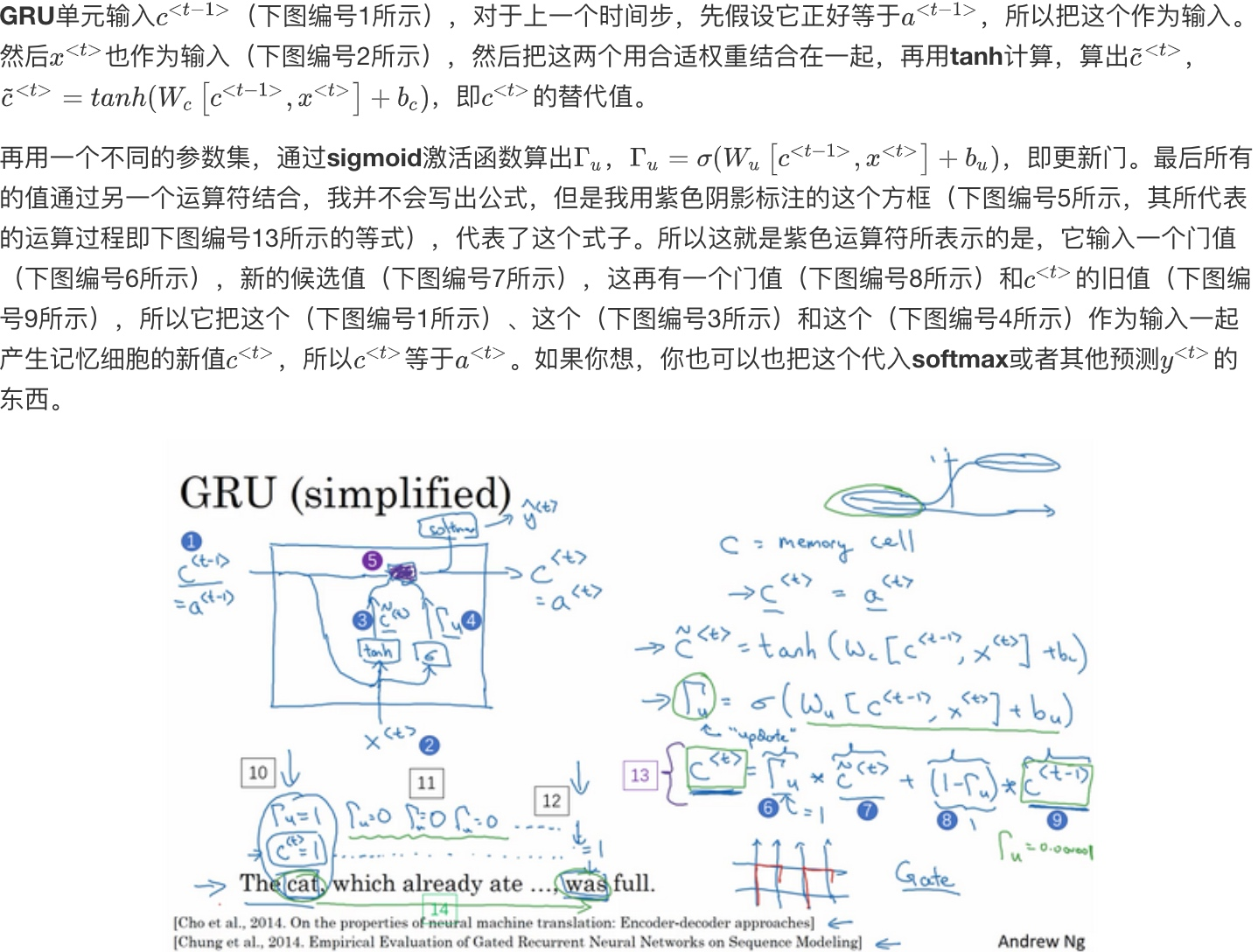GRU单元的优点就是通过门决定是否某个记忆细胞.

• $c^{<t>}$可以是一个向量.
• 假设有100维的隐藏的激活值, 那么$c^{<t>}, \tilde{c}^{<t>}, \Gamma_u$, 也是100维, 他们具有相同维度
• $\Gamma_u$是100维的向量，里面的值几乎都是0或者1, 它告诉了100维的记忆细胞$c^{<t>}$哪些是要更新的bit.
• 比如说你可能需要一个比特来记忆猫是单数还是复数，其他比特来理解你正在谈论食物，然后你稍后可能就会谈论“The cat was full.”，你可以每个时间点只改变一些bit

Full GRU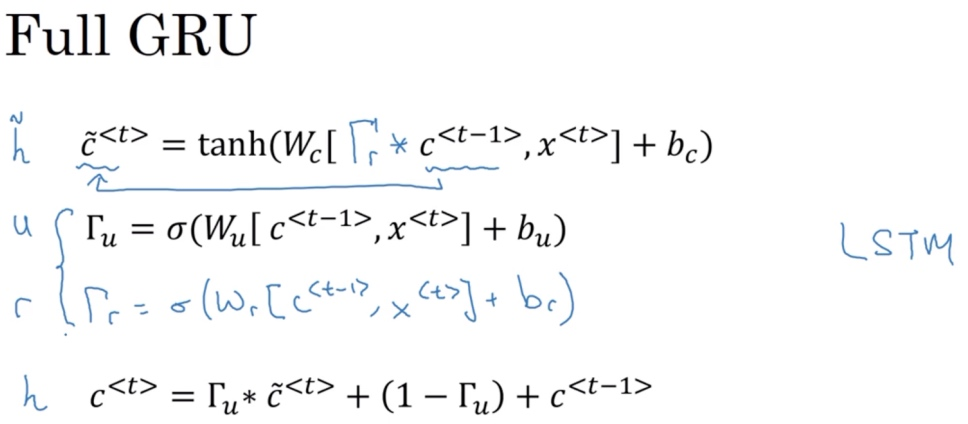## LSTM单元（long short term memory unit)

[Hochreiter S, Schmidhuber J. Long Short-Term Memory[J]. Neural Computation, 1997, 9(8):1735-1780.]

LSTM即长短时记忆网络, 比GRU更加有效.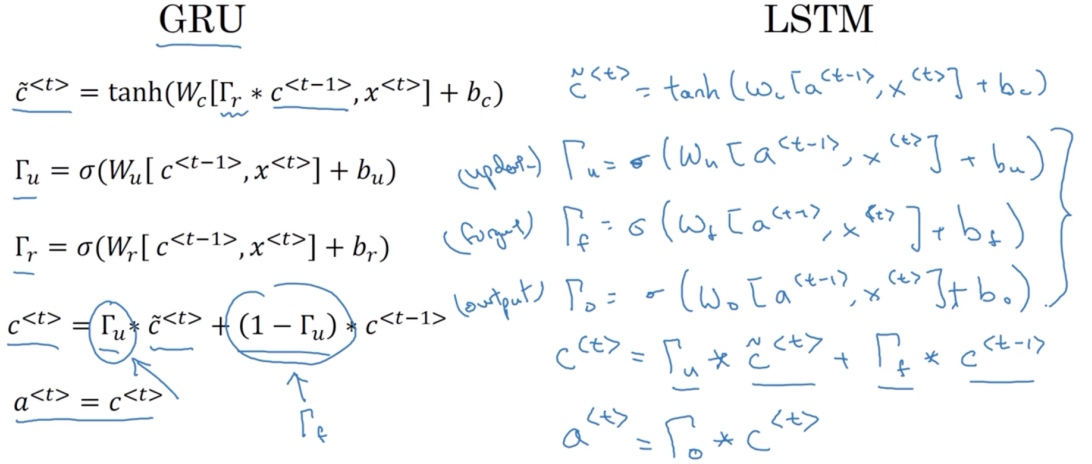LSTM的公式与GRU的不同:

• 候选值$\tilde{c}^{[t]}$公式中, 使用$a^{[t-1]}$代替$c^{[t-1]}$, 因此在LSTM中不再有的情况$a^{[t]}= c^{[t]}$
• 不再使用相关门$\Gamma_r$
• 多了遗忘门$\Gamma_f$(the forget gate) 和 输出门$\Gamma_o$(output gate)
• 记忆细胞的更新值 $c^{[t]} = \Gamma_u * \tilde{c}^{[t]} + \Gamma_f * c^{[t-1]}$, 给了记忆细胞选择权去维持旧的值$c^{[t-1]}$或者就加上新的值$\tilde{c}^{[t]}$
• 最后$a^{[t]} = \Gamma_o * c^{[t]}$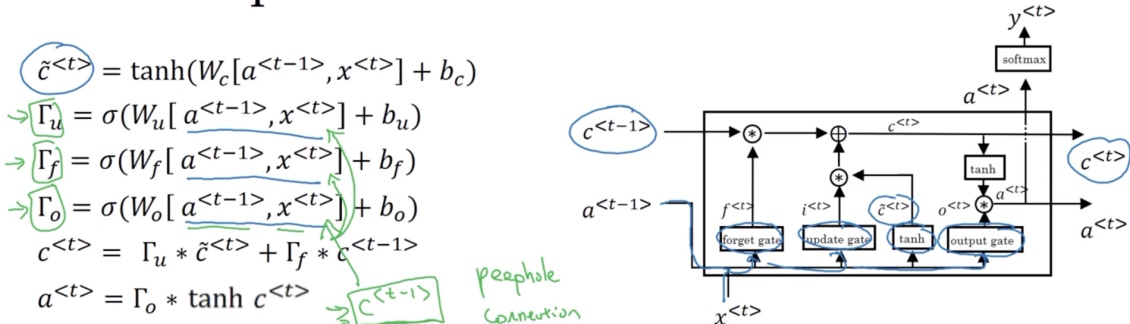LSTM另外一些版本可能门值不仅取决于$a^{<t-1>}, x^{<t>}$, 有时候也可以偷窥(取决)一下上一个记忆细胞的值$c^{<t-1>}$, 这叫做”窥视孔连接”(peephole connection). 后“偷窥孔连接”就可以结合这三个门（$\Gamma_u,\Gamma_f,\Gamma_o$）来计算了. 第n个$c^{<t-1>}$的元素只会影响第n个元素对应的那个门.

LSTM前向传播图: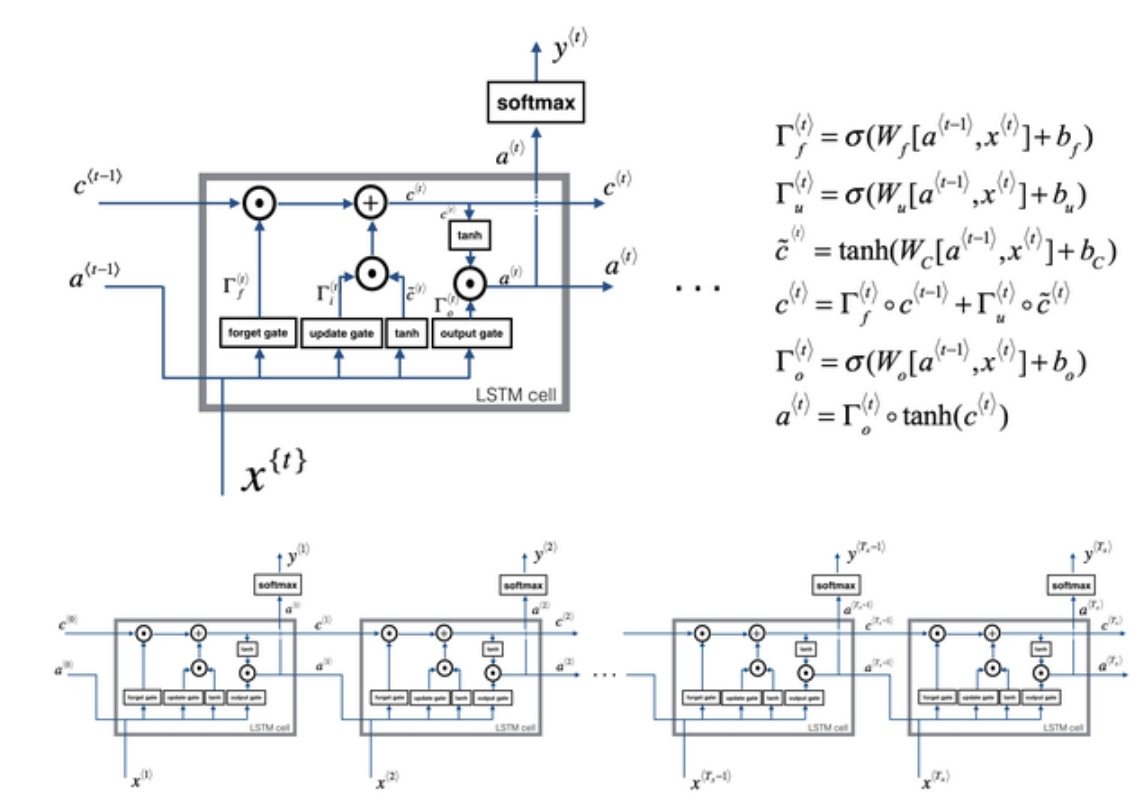LSTM反向传播计算: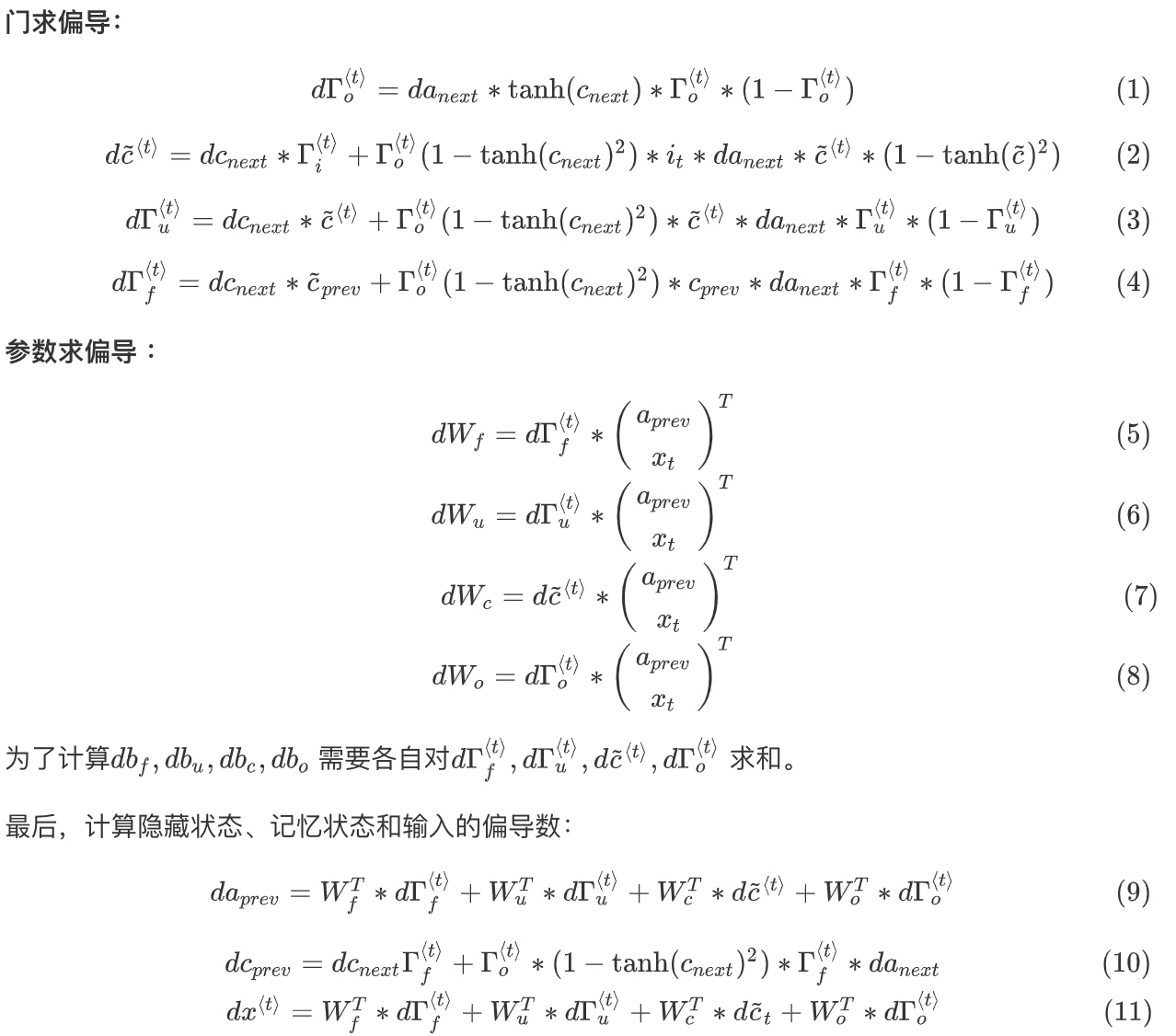GRU的优点是这是个更加简单的模型，所以更容易创建一个更大的网络，而且它只有两个门，在计算性上也运行得更快，然后它可以扩大模型的规模

LSTM更加强大和灵活，因为它有三个门而不是两个

## 双向循环神经网络（Bidirectional RNN）

He said, ‘Teddy bears are on sales’

He said, ‘Teddy Roosevelt was a good man’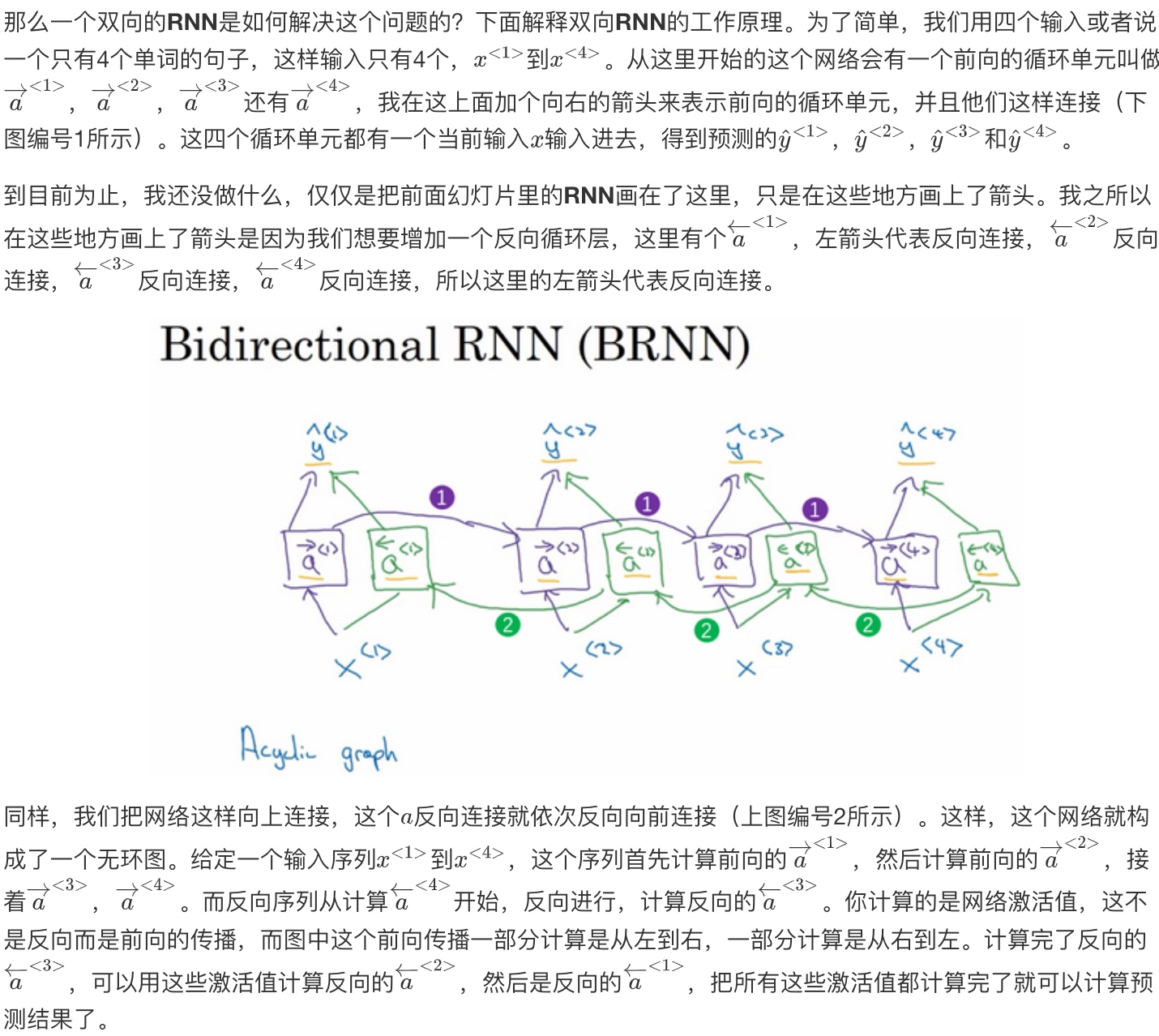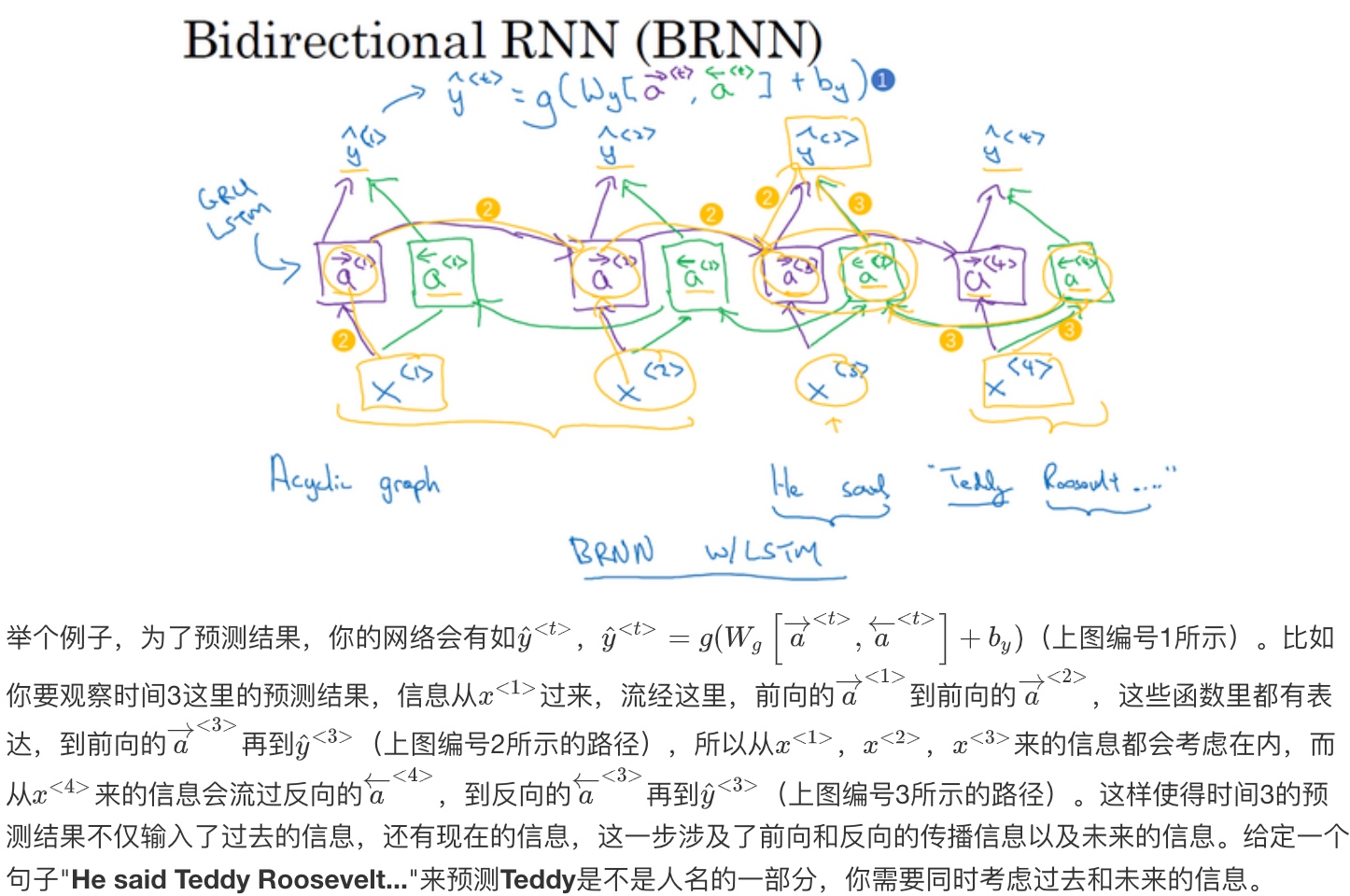## 深层循环神经网络（Deep RNNs）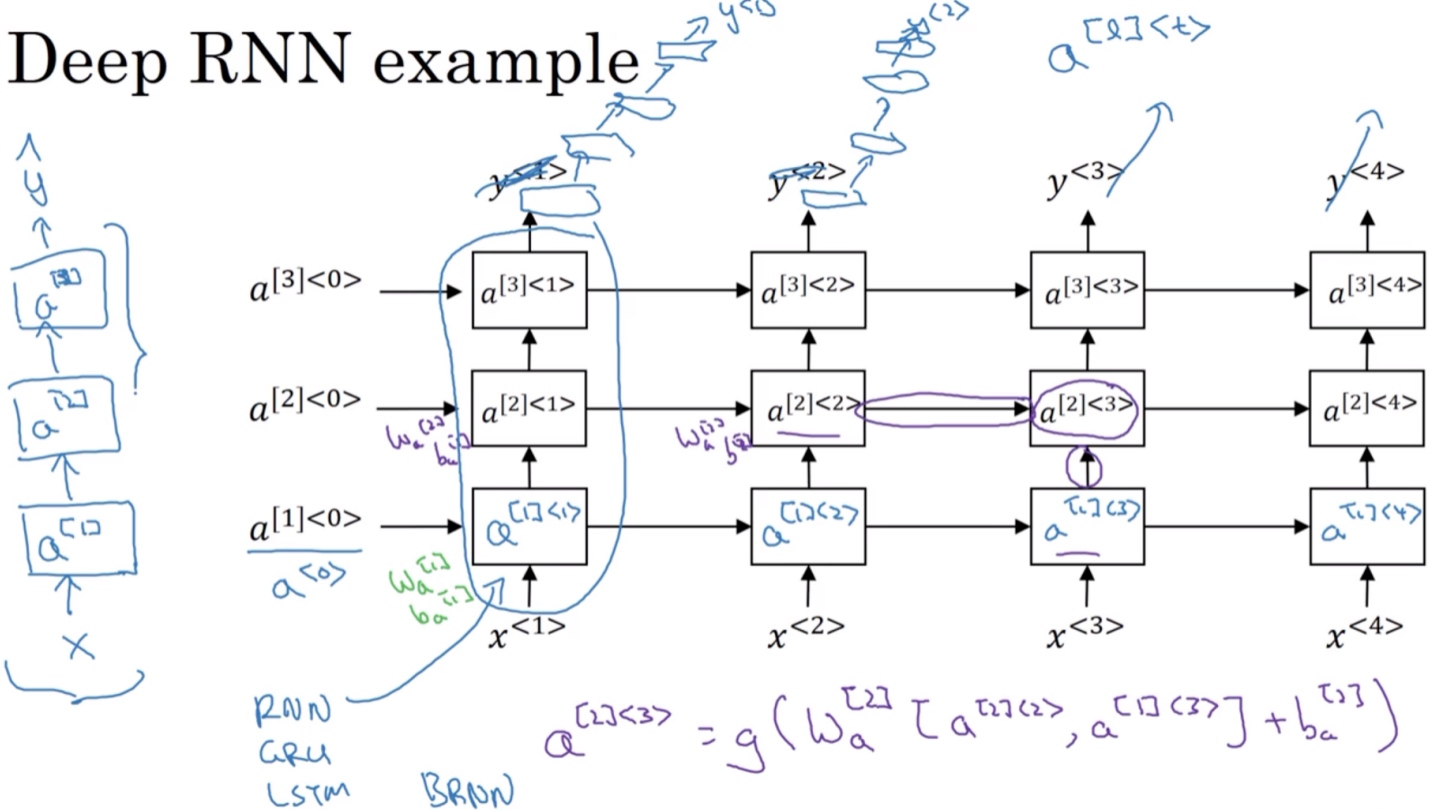$a^{<1>}$表示第一层第一个时间点的激活值.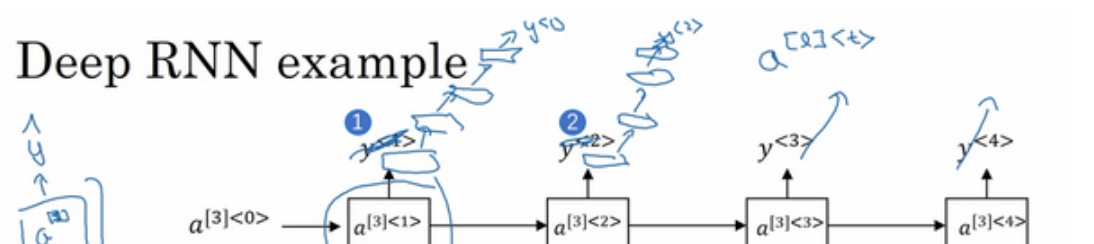Ref: